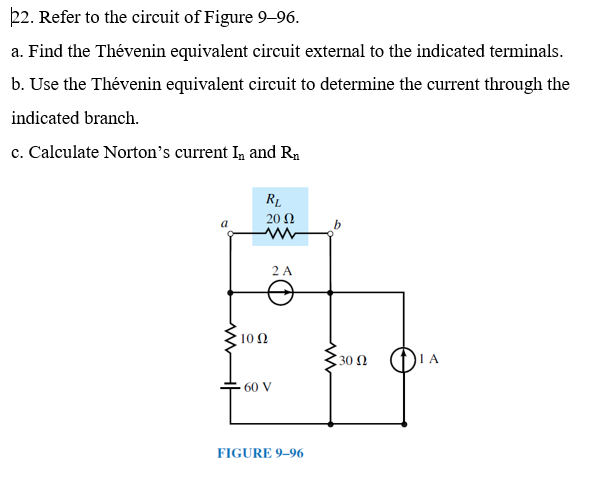# Question Solved1 Answer22. Refer to the circuit of Figure 9–96. a. Find the Thévenin equivalent circuit external to the indicated terminals. b. Use the Thévenin equivalent circuit to determine the current through the indicated branch. c. Calculate Norton's current I, and Rn RL 20 Ω 2 A 1012 w 30 12 60 V FIGURE 9-96M6XQHC The Asker · Electrical EngineeringTranscribed Image Text: 22. Refer to the circuit of Figure 9–96. a. Find the Thévenin equivalent circuit external to the indicated terminals. b. Use the Thévenin equivalent circuit to determine the current through the indicated branch. c. Calculate Norton's current I, and Rn RL 20 Ω 2 A 1012 w 30 12 60 V FIGURE 9-96
More
Transcribed Image Text: 22. Refer to the circuit of Figure 9–96. a. Find the Thévenin equivalent circuit external to the indicated terminals. b. Use the Thévenin equivalent circuit to determine the current through the indicated branch. c. Calculate Norton's current I, and Rn RL 20 Ω 2 A 1012 w 30 12 60 V FIGURE 9-96